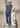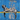# Sum of TwoMarch 05, 2020

This is the infamous Google coding interview question. SUM OF TWO!!!

## The Problem:

You have two integer arrays, a and b, and an integer target value v. Determine whether there is a pair of numbers, where one number is taken from a and the other from b, that can be added together to get a sum of v. Return true if such a pair exists, otherwise return false.

For example,

``````a = \[1, 2, 3]\
b = \[10, 20, 30, 40]\
v = 42\
40 + 2 = 42\
sumOfTwo(a, b, v) = true``````

Use your intuition to come up with an algorithm.

### Solution 1 — Brute Force Method

Use as many nested for loops as necessary, big O time be damned! Here’s the brute force beast solution:

``````// Brute Force Method

let array1 = [5, 2, 1];
let array2 = [5, 2, 3];
let targetValue = 10;
let pairExists = false;
let arr1Val = -1;
let arr2Val = -1;

function sumOfTwo(arr1, arr2, tarVal) {
for (var i = 0; i < arr1.length; i++) {
var needed_value = tarVal - arr1[i];
for (var x = 0; x < arr2.length; x++) {
if (arr2[x] === needed_value) {
pairExists = true;
arr1Val = arr1[i];
arr2Val = arr2[x];
break;
}
}
}
}

sumOfTwo(array1, array2, targetValue);
console.log(`Array 1 : \${array1}`);
console.log(`Array 2 : \${array2}`);
console.log("Pair exists = " + pairExists);
console.log("Array 1 Value = " + arr1Val);
console.log("Array 2 Value = " + arr2Val);``````

YIKES! I mean, it works. But it’s about as inefficient as possible. We can do better.

### Solution 2 — Hash Set Method

When you want to go faster when programming, use data structures! In this case, a hash set! Or rather, since this is JavaScript, a hash set object! Here it is:

``````// Hash Set Object Data Structure Method -- Much Better

let array1 = [5, 2, 1];
let array2 = [2, 2, 3, 4, 1, 7];
let targetValue = 10;

let pairExists = false;
let arr1Val = -1;
let arr2Val = -1;

var hash = {};

var checkValue = function(value) {
return hash[value] === true;
};

function sumOfTwo(arr1, arr2, tarVal) {
for (var i = 0; i < arr1.length; i++) {
var difference = tarVal - arr1[i];
hash[difference] = true;
}
console.log(hash);
for (var x = 0; x < arr2.length; x++) {
if (checkValue(arr2[x])) {
pairExists = true;
arr1Val = tarVal - arr2[x];
arr2Val = arr2[x];
}
break;
}
}

sumOfTwo(array1, array2, targetValue);
console.log(`Array 1 : \${array1}`);
console.log(`Array 2 : \${array2}`);
console.log("Pair exists = " + pairExists);
console.log("Array 1 Value = " + arr1Val);
console.log("Array 2 Value = " + arr2Val);``````

The way it works is on the first for loop you iterate through the first array and for each element you calculate the needed value to sum to the target value, and then you log that needed value in the hash set object. Then, once the for loop is done and it has filled up the has set, you start the next for loop and for each element check to see if it’s listed in the hash set object with a ‘true’ value, indicating that it is a pair!

This way we can have two sequential for loops instead of a nested for loop. If the brute force method is “A x B”, this hash set data structure method is “A + B”. MUCH BETTER!

The moral of the story: use data structures!

Okay, can I get that Google job now, pls. thx :-P

-mrWritten by Matthew Riddett who lives and works in Victoria BC, building fun and useful things. You can follow him on Twitter